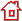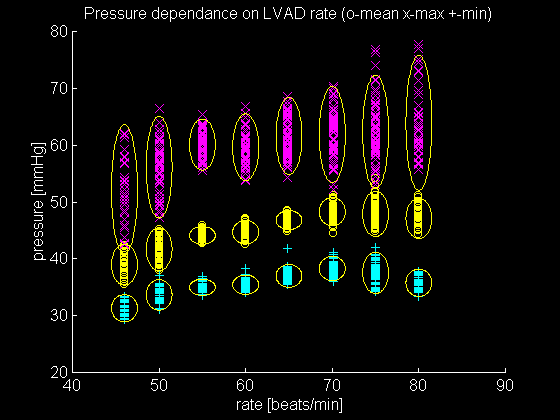# Pressure-rate relationship

The following graph shows dependance of the arterial pressure on the LVAD rate. For each heart beat, 3 points are plotted: (+) denoting minimal, (x) maximal and (o) mean pressure. The 3 points for each beat are plotted in the column corresponding to the LVAD rate.

The ellipses are centered at the mean value of a set of points for a constant LVAD rate, and have the hight (greated diameter) of four standard deviations for the set of points. So, for each of eight different LVAD rates, 3 ellipses encircle points of peak, minimal and mean pressure.